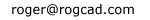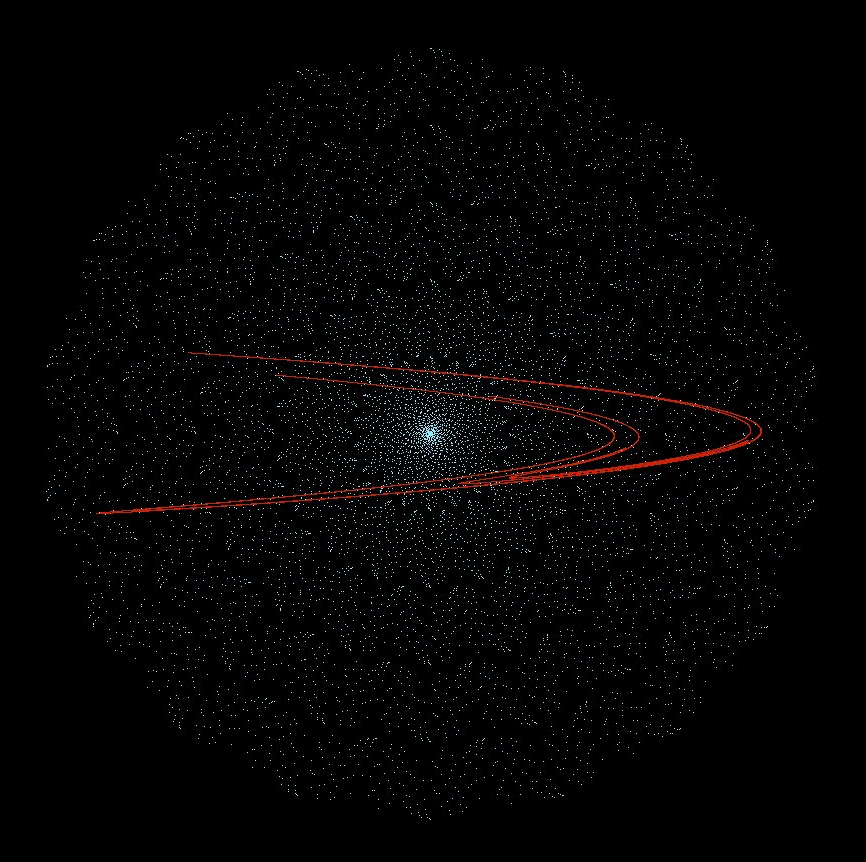```----------------

home

Balloting

Simple trig

Logistic map

The Attractor of Henon

Number doubling

Barnsley's Fern

The Sierpinski Triangle

The Attractor of Henon

X = ((previousY + 1) - (1.4 * previousX ^ 2))
Y = (0.3 * previousX)

"Iterating this pair of equations produces a strange
simple set of curved lines that is poorly understood
by mathematicians.  As thousands, then millions of
points appear, more and more detail emerges.  What
appear to be single lines prove, on magnification,
to be pairs, then pairs of pairs, and so on to
infinity.  Whether any two successive points appear
nearby or far apart is unpredictable." - James Gleick

I used .3, .2 as initial values for X, Y
(built into the program code)

then 60,
then 100,
then 200,
then 400,
then 800,
then 1500,
then 3000,
then 10000
then 20000
then 40000
then 100000

watching the strange attractor slowly materialize.For fun, the attractor superimposed onto a starfield
(the "double a number" algorithm)Here is my VisualBASIC program code:

Private Sub attractorbtn_Click()

ITER = Val(itera.Text)

OLDX = 0.3: OLDY = 0.2

For ATTR = 1 To ITER

X = ((OLDY + 1) - (1.4 * OLDX ^ 2))
Y = (0.3 * OLDX)

TEMPX = (260 * X) + 430
TEMPY = (260 * Y) + 530

viewport.PSet (TEMPX, TEMPY), RGB(208, 32, 6)

OLDX = X
OLDY = Y

Next ATTR

End Sub

=============================================================

home

Balloting

Simple trig

Logistic map

The Attractor of Henon

Number doubling

Barnsley's Fern

The Sierpinski Triangle

```